## Tuesday, 4 January 2011

### A Nice Property of the Medians of a Triangle

We begin with a simple triangle, ABC, with opposite sides a, b, and c; and median AD which we will call m.The object of the exercise; to find the length of the median, m, given the length of the three sides, a, b, and c.
We begin by asserting a known, and easy to prove, theorem of parallelograms. The sum of the squares of the sides of a parallelogram is equal to the sum of the squares of the diagonals. The proof follows easily from the use of the law of cosines and the fact that the cosines of adjacent angles of the parallelogram are opposites of each other, one positive and one negative.

But how, you may ask, do parallelograms figure in the argument. If we make a congruent copy of ABC and rotate it 180 degrees around D, the midpoint of segment c we get a parallelogram and its two diagonals.We observe that the diagonal AA’ has length 2m, and the other diagonal has length a. From the previously stated theorem it is then clear that :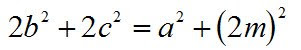Which leads simply to the result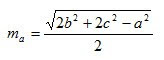The fact that this result was known before the birth of Christ does not diminish the simple elegance of the derivation.
To find the other two medians lengths, of course, it is only necessary to rotate the variables a, b, and c,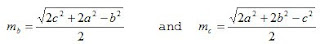.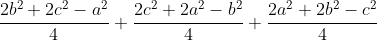.

Now collecting like terms we get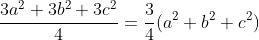.

And we see that in any triangle, the sum of the squares of the medians is three-fourths the sum of the squares of the sides. Pretty!Anonymous said...

There's a typo in the next to last formula. In the last term a and c need to be swapped.

JonathanAnonymous said...

oops. make that b and c.

Pat's Blog said...

Thanks JD, You'd think a college grad could do a simple cyclic permutation of three letters...
Really I think OK, but I type crappy.
Thanks again for the corrections